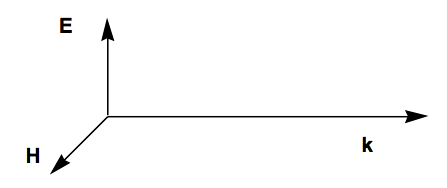Skip to main content

# 14.1: Time-Dependent Vector Potentials

•• Contributed by Jack Simons
• Professor Emeritus and Henry Eyring Scientist (Chemistry) at University of Utah

The full N-electron non-relativistic Hamiltonian H discussed earlier in this text involves the kinetic energies of the electrons and of the nuclei and the mutual Coulombic interactions among these particles

$H = \sum\limits_{a=1,M} -\left(\dfrac{\hbar^2}{2m_a}\right) \nabla_a^2 + \sum\limits_j \left[ \left(-\dfrac{\hbar^2}{2m_e}\right) \nabla_j^2 - \sum\limits_a Z_a \dfrac{e^2}{r_{j,a}} \right] + \sum\limits_{j<k}\dfrac{e^2}{r_{j,k}} + \sum\limits_{a<b}Z_aZ_b \dfrac{e^2}{R_{a,b}}.$

When an electromagnetic field is present, this is not the correct Hamiltonian, but it can be modified straightforwardly to obtain the proper H.

### The Time-Dependent Vector $$\textbf{A}(\textbf{r},t)$$ Potential

The only changes required to achieve the Hamiltonian that describes the same system in the presence of an electromagnetic field are to replace the momentum operators P$$_a$$ and p$$_j$$ for the nuclei and electrons, respectively, by (P$$_a$$ - Z$$_a$$ e/c A(R$$_a$$,t)) and (p$$_j$$ - e/c A(rj ,t)). Here Za e is the charge on the ath nucleus, -e is the charge of the electron, and c is the speed of light.

The vector potential A depends on time t and on the spatial location r of the particle in the following manner:

$\textbf{A}(\textbf{r},t) = 2 \textbf{A}_0 cos(\omega t - \textbf{k}\cdot{\textbf{r}}).$

The circular frequency of the radiation $$\omega$$ (radians per second) and the wave vector k (the magnitude of k is |k| = $$\frac{2\pi}{\lambda}$$, where $$\lambda$$ is the wavelength of the light) control the temporal and spatial oscillations of the photons. The vector $$\textbf{A}_o$$ characterizes the strength (through the magnitude of $$\textbf{A}_o$$) of the field as well as the direction of the A potential; the direction of propagation of the photons is given by the unit vector k/|k|. The factor of 2 in the definition of A allows one to think of $$\textbf{A}_0$$ as measuring the strength of both $$e^{i(\omega t - \textbf{k}\cdot{\textbf{r}})}$$ and $$e^{i(\omega t - \textbf{k}\cdot{\textbf{r}})}$$ components of the $$cos(\omega t - \textbf{k}\cdot{\textbf{r}})$$ function.

## The Electric $$\textbf{E}(\textbf{r},t) \text{ and Magnetic } \textbf{H}(\textbf{r},t) \text{ Fields }$$

The electric $$\textbf{E}(\textbf{r},t) \text{ and magnetic } \textbf{H}(\textbf{r}$$,t) fields of the photons are expressed in terms of the vector potential A as

$\textbf{E}(\textbf{r},t) = -\dfrac{1}{3}\dfrac{\partial \textbf{A}}{\partial t} = \dfrac{\omega}{c}\textbf{A}_0 \sin( \omega t - \textbf{k}\cdot{\textbf{r}} )$

$\textbf{H}(\textbf{r},t) = \nabla \textbf{ x A } = \textbf{ k x A}_o 2 \sin(\omega t - \textbf{k}\cdot{\textbf{r}}).$

The E field lies parallel to the $$\textbf{A}_o$$ vector, and the H field is perpendicular to $$\textbf{A}_o$$; both are perpendicular to the direction of propagation of the light k/|k|. E and H have the same phase because they both vary with time and spatial location as $$\sin (\omega t - \textbf{k}\cdot{\textbf{r}}).$$ The relative orientations of these vectors are shown below.Figure 14.1.1: Insert caption here!

## The Resulting Hamiltonian

Replacing the nuclear and electronic momenta by the modifications shown above in the kinetic energy terms of the full electronic and nuclear-motion hamiltonian results in the following additional factors appearing in H:

$H_{int} = \sum\limits_j \left[ \dfrac{ie\hbar}{m_ec}\textbf{A}(r_j,t)\cdot{\nabla_j} + \left( \dfrac{e^2}{2m_ec^2} \right)|\textbf{A}(r_j,t)|^2 \right] + \sum\limits_a \left[ \left( iZ_a\dfrac{e\hbar}{m_ac} \right)\textbf{A}(R_a,t)\cdot{\nabla_a} + \left( \dfrac{Z_a^2e^2}{2m_ac^2} \right)|\textbf{A}(R_a,t)|^2 \right].$

These so-called interaction perturbations $$H_{int}$$ are what induces transitions among the various electronic/vibrational/rotational states of a molecule. The one-electron additive nature of $$H_{int}$$ plays an important role in determining the kind of transitions that $$H_{int}$$ can induce. For example, it causes the most intense electronic transitions to involve excitation of a single electron from one orbital to another (e.g., the Slater-Condon rules).